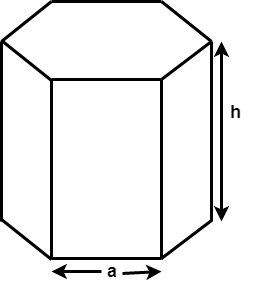# Surface Area and Volume of Hexagonal Prism

Given a Base edge and Height of the Hexagonal prism, the task is to find the Surface Area and the Volume of hexagonal Prism. In mathematics, a hexagonal prism is a three-dimensional solid shape which have 8 faces, 18 edges, and 12 vertices. The two faces at either ends are hexagons, and the rest of the faces of the hexagonal prism are rectangular.where a is the base length and h is the height of the hexagonal prism.

Surface Area =Volume =Examples:

Input : a = 4, h = 3
Output : Surface Area: 155.138443
Volume: 124.707657

Input : a = 5, h = 10
Output : Surface Area: 429.904
Volume: 649.519


## C++

 // C++ program to find the Surface Area  // and Volume of Hexagonal Prism.     #include  using namespace std;     // Function to calculate Surface area  void findSurfaceArea(float a, float h)  {      float Area;         // Formula to calculate surface area      Area = 6 * a * h + 3 * sqrt(3) * a * a;         // Display surface area      cout << "Surface Area: " << Area;      cout << "\n";  }     // Function to calculate Volume  void findVolume(float a, float h)  {      float Volume;         // formula to calculate Volume      Volume = 3 * sqrt(3) * a * a * h / 2;         // Display Volume      cout << "Volume: " << Volume;  }     // Driver Code  int main()  {      float a = 5, h = 10;             // surface area function call      findSurfaceArea(a, h);         // volume function call      findVolume(a, h);         return 0;  }

## Java

 // Java program to find the Surface Area  // and Volume of Hexagonal Prism.     import java.io.*;     class GFG {                // Function to calculate Surface area      static void findSurfaceArea(float a, float h)      {          float Area;             // Formula to calculate surface area          Area = 6 * a * h + 3 * (float)(Math.sqrt(3)) * a * a;                 // Display surface area          System.out.println("Surface Area: " + Area);      }             // Function to calculate Volume      static void findVolume(float a, float h)      {          float Volume;                 // formula to calculate Volume          Volume = 3 * (float)(Math.sqrt(3)) * a * a * h / 2;                 // Display Volume          System.out.println("Volume: " + Volume);      }         // Driver code      public static void main (String[] args)      {          float a = 5, h = 10;                 // surface area function call          findSurfaceArea(a, h);                 // volume function call          findVolume(a, h);      }  }

## Python3

 # Python3 program to find the   # Surface Area and Volume  # of Hexagonal Prism.  import math     # Function to calculate  # Surface area  def findSurfaceArea(a, h):      Area = 0;         # Formula to calculate      # surface area      Area = (6 * a * h +              3 * math.sqrt(3) * a * a);         # Display surface area      print("Surface Area:",             round(Area, 3));         # Function to   # calculate Volume  def findVolume(a, h):      Volume = 0;         # formula to       # calculate Volume      Volume = (3 * math.sqrt(3) *                  a * a * h / 2);         # Display Volume      print("Volume:",              round(Volume, 3));     # Driver Code  a = 5;   h = 10;     # surface area  # function call  findSurfaceArea(a, h);     # volume function call  findVolume(a, h);     # This code is contributed   # by mits

## C#

 // C# program to find the  // Surface Area and Volume  // of Hexagonal Prism.  using System;     class GFG   {                 // Function to calculate      // Surface area      static void findSurfaceArea(float a,                                   float h)      {          float Area;             // Formula to calculate           // surface area          Area = 6 * a * h + 3 *                  (float)(Math.Sqrt(3)) * a * a;                 // Display surface area          Console.WriteLine("Surface Area: " +                                         Area);      }             // Function to      // calculate Volume      static void findVolume(float a,                              float h)      {          float Volume;                 // formula to calculate Volume          Volume = 3 * (float)(Math.Sqrt(3)) *                                 a * a * h / 2;                 // Display Volume          Console.WriteLine("Volume: " +                                 Volume);      }         // Driver code      public static void Main ()      {          float a = 5, h = 10;                 // surface area          // function call          findSurfaceArea(a, h);                 // volume function call          findVolume(a, h);      }  }     // This code is contributed   // by anuj_67.

## PHP

 

Output:

Surface Area: 429.904
Volume: 649.519


Attention reader! Don’t stop learning now. Get hold of all the important DSA concepts with the DSA Self Paced Course at a student-friendly price and become industry ready.

My Personal Notes arrow_drop_upCheck out this Author's contributed articles.

If you like GeeksforGeeks and would like to contribute, you can also write an article using contribute.geeksforgeeks.org or mail your article to contribute@geeksforgeeks.org. See your article appearing on the GeeksforGeeks main page and help other Geeks.

Please Improve this article if you find anything incorrect by clicking on the "Improve Article" button below.

Improved By : vt_m, Mithun Kumar